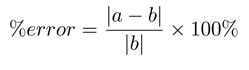SEARCH HOMEMath Central Quandaries & QueriesQuestion from Bill: If say, a prediction is 20 and the actual is 35 how do you work out the accuracy of the predictionHi Bill,

Percent Error is calculated by determining an experimental value (b) from your results and then comparing it to the accepted literature value (a) according to the formula:In your case, the experimental value is 35 accepted value would be 20. Some things to consider when calculating accuracy:

• Is your prediction based on a known or accepted value?
• Is our experimental value based on an average from many trials of an experiment?

If these above conditions are not met then the percent error is uncertain. Accuracy can be expressed as

100%-percent error

Hope this helps,

JaniceMath Central is supported by the University of Regina and The Pacific Institute for the Mathematical Sciences.